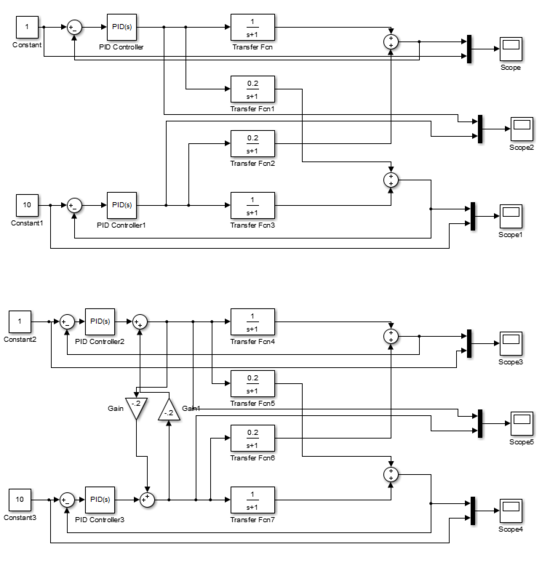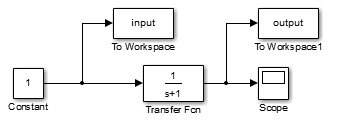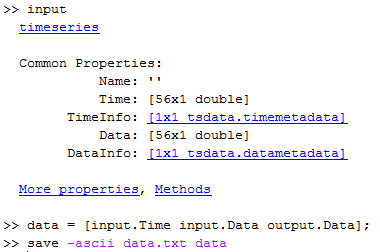## Simulation Lab Programming Help

There are several online courses and materials that can help students gain basic programming experience in MATLAB or Python. Below are some online resources:

Below are a few examples of Simulink and MATLAB programs that can help with projects. Common questions for the simulation labs include how to import data, export results, and configuring more complex systems.

### Import Data File into Simulink

Data such as any custom time series can be imported into Simulink. In this example, an Interpreted MATLAB Function block is used to read a data file (data.txt) and interpolate with a linear approximation between the two nearest points.

### Example Multiple-Input, Multiple-Output (MIMO) System

The following Simulink model is an example of a Multiple-Input Multiple-Output system. The first block is an example of two decoupled PID controllers (non-interacting) while the second block is an example of interacting PID controllers with feedforward gains.### Exporting Data from MATLAB / Simulink

You may want to export data from MATLAB or Simulink to combine plots or for further manipulation in another program like Excel. One way is shown below:

Step 1: Drop the "to Workspace" blocks into Simulink and re-run the simulation. The requested variables will be exported to the MATLAB workspace.Step 2: From the MATLAB command prompt, view the "input" and "output" data that was generated from the Simulink simulation. Type "input" or "output" to inspect the information that is contained in the variable structure.Step 3: Save the data to a text file. One way is to construct all of the data that will be saved into a data matrix and then export as an ASCII text file (tab delimited). Make sure your current directory allows you to save a file (is not write-restricted) or it will return an error.